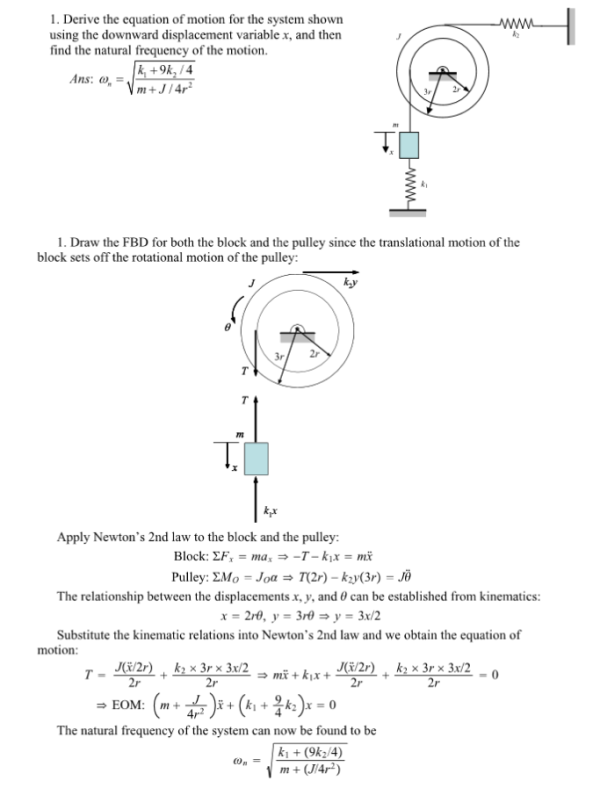Derive the equation of motion for the system shown using the downward displacement variable x, and then find the natural frequency of the motion.Derive the equation of motion for the system shown using the downward displacement variable x, and then find the natural frequency of the motion.

System Dynamics Page 2 dynamics dynamics dynamics dynamics dynamics dynamics dynamics System dynamics Page 3